CATEGORIES:

# As a result, we obtain;.

By definition,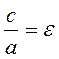is the eccentricity of the hyperbola; we have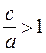, because с>а.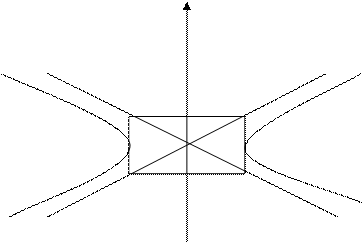By analogy with an ellipse, the directrices of a hyperbola are determined by the equationsand.

y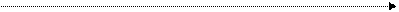M1(–а;0) 0 M2(а;0) x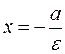Since the eccentricity of a hyperbola is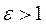, it follows that the directrix is; i.e., the directrices lie between the two vertices.

The ratios of the focal radii to the corresponding distances from an arbitrary point of the hyperbola to the directrices is constant and equals the eccentricity:.

Tangent lines to a hyperbola. Suppose given, the equation of a hyperbola and a point М000) on it: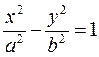.

As is known, the equation of a tangent to a curve is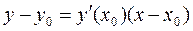.

Let us differentiate the equation of a hyperbola as an implicit function: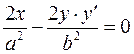; we obtain, or.

Substituting, we obtain the equation of the tangent line:,

Let us transform it: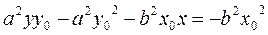;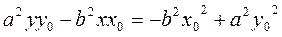. Dividing by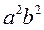, we obtain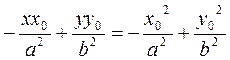.

Since the point М0 belongs to the hyperbola, its coordinates must satisfy the equation of the hyperbola, and, the right-hand side equals (–1) .

Thus, the equation of a tangent to a hyperbola is.

Example. Write the equation of the hyperbola with real semi axis 4 and foci at the points F1(5;0) and F2(5;0).

Formula (19) gives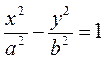We have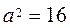and; thus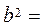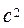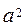=25–16=9.

The required classical equation of the hyperbola is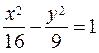.

Date: 2015-01-02; view: 831

 <== previous page | next page ==> Asymptotes of a Hyperbola | Parabola
doclecture.net - lectures - 2014-2022 year. Copyright infringement or personal data (0.021 sec.)